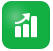## Setting the Calculation Logic of Demand Drivers

On the Demand Driver Data tab, you set the demand calculation logic and the values of the demand drivers for each year.

To set the calculation logic for a driver that calculates the Demand FTE:

1. Click Demand.
2. Click Demand Driver Data, and under Calculation Logic, select one of the following:

• Previous Year’s Ratio: Bases the demand on the previous year’s demand rate and applies the Demand Scale value if Demand Driver Scaling Factor is enabled and scaling is set. If no calculation logic is specified, then Previous Year's Ratio is applied. See Scaling Demand FTE and Factoring Productivity Changes into Demand FTE.

• Demand = Driver / Rate: Derives demand by dividing the driver by the rate. For example, for every \$5 million in revenue, you need one partner.

• Demand = Driver * Rate: Derives demand by multiplying the driver by the rate. For example, multiply processing units by cost.

• Custom: Derives demand using a custom member formula on the dynamically calculated member Demand FTE Custom. If the predefined calculation logic doesn't fit your needs, your administrator can create a custom member formula using the Dimension Editor.

For example, here's a simple member formula that returns the Demand FTE as 52:

`IF ("OWP_Calculation Logic"->"OWP_Headcount Driver"->"No Year"->"Begbalance" == [OWP_Demand_CalcLogic.Custom]) "OWP_Demand FTE"->"No Property" = 52; ENDIF;`

Note:

Refer to the OWP_Demand FTE account member in the formula because that member is used in forms. The member Demand FTE Custom isn't used in forms. Also, ensure that the formula is appropriate and doesn't impact performance.

3. Under Demand Units, enter the demand units (for example, the number of insurance policies or the number of revenue units).

4. Enter a value into Demand Scale to increase or decrease the calculated Demand FTE when using the Previous Year’s Ratio calculation logic.

If your administrator enabled the option Demand Driver Scaling Factor, you can use Demand Scale to scale your demand planning to account for a change in productivity over time. In calculating the future demand for FTE, demand scaling factors in natural logarithm as an exponent of the ratio. If you don’t enter a Demand Scale value, the demand is assumed to be 100% of the demand driver. The demand scale applies to all jobs in the entity, regardless of whether the demand driver is based on Entity or Job. If a driver's calculation logic is not set to Previous Year's Ratio and you enter a value into Demand Scale, the value won't impact the demand calculations. (Custom calculation logic might be an exception to this rule, depending on how the formula is defined.) See Scaling Demand FTE.

5. Enter a value into Expected Productivity to further refine FTE calculations.

If your administrator enabled Expected Productivity Gains Factor, you can adjust FTE calculations by setting a value in Expected Productivity. Regardless of the calculation logic selected, Expected Productivity is factored into Demand FTE calculations if Expected Productivity Gains Factor is enabled and a value entered. See Factoring Productivity Changes into Demand FTE.